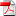# Some machine learning schemes for high-dimensional nonlinear PDEs - C. HURE, H. PHAM, X. WARIN

26
Fév

We propose new machine learning schemes for solving high dimensional nonlinear partial differential equations (PDEs). Relying on the classical backward stochastic differential equation (BSDE) representation of PDEs, our algorithms estimate simultaneously the solution and its gradient by deep neural networks. These approximations are performed at each time step from the minimization of loss functions de ned recursively by backward induction. The methodology is extended to variational inequalities arising in optimal stopping problems. We analyze the convergence of the deep learning schemes and provide error estimates in terms of the universal approximation of neural networks. Numerical results show that our algorithms give very good results till dimension 50 (and certainly above), for both PDEs and variational inequalities problems. For the PDEs resolution, our results are very similar to those obtained by the recent method in [EHJ17] when the latter converges to the right solution or does not diverge. Numerical tests indicate that the proposed methods are not stuck in poor local minima as it can be the case with the algorithm designed in [EHJ17], and no divergence is experienced. The only limitation seems to be due to the inability of the considered deep neural networks to represent a solution with a too complex structure in high dimension.

•RR-FIME -19-01